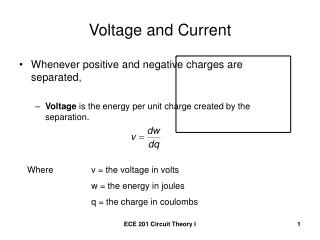# Voltage and Current - PowerPoint PPT PresentationDownload PresentationVoltage and Current

Voltage and CurrentDownload Presentation## Voltage and Current

- - - - - - - - - - - - - - - - - - - - - - - - - - - E N D - - - - - - - - - - - - - - - - - - - - - - - - - - -
##### Presentation Transcript

1. Voltage and Current • Whenever positive and negative charges are separated, • Voltage is the energy per unit charge created by the separation. Where v = the voltage in volts w = the energy in joules q = the charge in coulombs ECE 201 Circuit Theory I

2. Voltage and Current (continued) • The rate of charge flow is electric current Where i = the current in amperes q = the charge in coulombs t = the time in seconds ECE 201 Circuit Theory I

3. Power and Energy • Power is the time rate of expending or absorbing energy. Where p = the power in watts w = the energy in joules t = the time in seconds 1 Watt = 1 Joule/second ECE 201 Circuit Theory I

4. Power Associated with the flow of charge • Using the definitions of Voltage and Current Where p = the power in Watts v = the voltage in volts i = the current in amperes ECE 201 Circuit Theory I

5. The Basic Ideal Circuit Element • The ideal basic circuit element • has only two terminals • is described mathematically in terms of current and/or voltage • Cannot be subdivided into other elements ECE 201 Circuit Theory I

6. Passive Sign Convention If the power is positive (p>0), power is being delivered to the circuit inside the box. If the power is negative (p<0), power is being extracted from the circuit inside the box. ECE 201 Circuit Theory I

7. Circuit Theory • What is an electric circuit? • A mathematical model that approximates the behavior of an actual electrical system. • Example: Automobile Headlights • Here the headlights are OFF and draw no current from the battery because the switch is open. ECE 201 Circuit Theory I

8. Automobile Headlights (continued) • Turn the headlights ON by closing the switch. The headlights are ON. They draw a current of 4.138 Amperes from the battery. ECE 201 Circuit Theory I

9. Jump Starting Example • The current is measured to be 40 Amperes. Which car has the dead battery? ECE 201 Circuit Theory I

10. The current is flowing into the + terminal of the battery in car A. p = vi = (12V)(40A) = 480W Since the power is positive, the battery in car A is absorbing power. Car A has the dead battery. ECE 201 Circuit Theory I

11. Additional Calculation • If this connection is maintained for 1.5 minutes, how much energy is transferred to the dead battery? ECE 201 Circuit Theory I